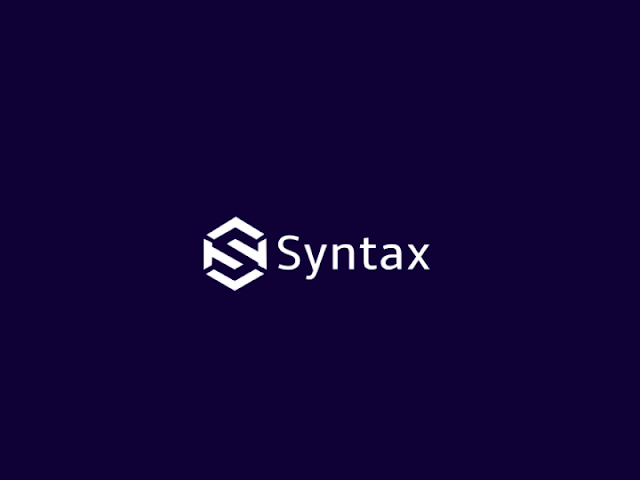# Midpoint Circle Drawing Algorithm

#include<stdio.h>

#include<conio.h>

#include<graphics.h>

int gdriver=DETECT,gmode;

void midpoint_circle();

void main(){

int xc=300;

int yc=200;

int r=100;

clrscr();

initgraph(&gdriver, &gmode, "C:\\Turboc3\\bgi");

midpoint_circle(xc, yc, r);

getch();

}

void midpoint_circle(int xc, int yc, int r){

int x=0;

int y=r;

int d=1-r;

draw_circle(xc, yc, x, y);

while(x<y){

x++;

if(d<0){

d=d+2*x+1;

}

else{

y--;

d=d+2*(x-y)+1;

}

draw_circle(xc, yc, x, y);

}

}

int draw_circle(int x0, int y0, int x, int y){

putpixel(x0+x, y0+y,2);

putpixel(x0+y, y0+x,2);

putpixel(x0-y, y0+x,2);

putpixel(x0-x, y0+y,2);

putpixel(x0-x, y0-y,2);

putpixel(x0-y, y0-x,2);

putpixel(x0+y, y0-x,2);

putpixel(x0+x, y0-y,2);

return 0;

}

Thick line program in C graphics

Armstrong program in Java with object